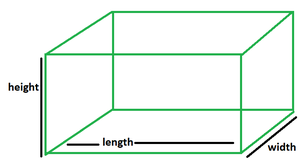GeeksforGeeks App
Open AppBrowser
Continue

# How to find the height of a cuboid when given the surface area?

Geometry and geometrical shapes play a pivotal role in our daily lives. Starting from a simple lunch box to a water tank, geometry surrounds us in different shapes and sizes. The shape and surface to be taken up by such shapes need to be calculated so as to store them without any difficulty, or to meet the needs of the people using them. Such study or computation is given a special name in the field of mathematics.

### What is Mensuration?

Whenever the dimensions of various geometrical shapes are computed and their area or capacity is measured in mathematics, it is termed mensuration.

Cuboid

Such a three-dimensional shape has 6 rectangular faces, 12 edges, and 8 vertices. It is convex in shape and not concave. As a 3D shape, it has a length, height, and width. In other words, when six rectangles are joined together in a certain manner, it forms a cuboid. In real life, we can see a lot of cuboidal shapes around us like tiffin boxes, books, shoebox, bricks, mattresses, etc.### Surface Area of Cuboid

• Total Surface Area: Since a cuboid is formed by joining 6 rectangles in a definite fashion, the area of a cuboid would be equal to the surface area of all the rectangles combined together. Alternatively, the following formula can be used to calculate the surface area of a cuboid with its length being l, width being w, and height denoted by h.
• Lateral Surface Area: It is obtained by finding the area of the given cuboid without including the area of the top and base. Thus, when the area of only the sideward faces is calculated, and not that of the top and the base, it amounts to the lateral surface area.

Formulas

Total surface area of a cuboid = 2(lb + bh + lh), where the products of lengths, breadths, heights represent the areas of two rectangles having identical dimensions.

Lateral surface area of a cuboid = 2h (l + b), where the products of lengths, breadths, heights represent the areas of two rectangles having identical dimensions.

### How do you find the height of a cuboid when given the surface area?

• Total Surface Area

Let the surface area be denoted by a, length, breadth and height with l, b, h respectively.

Hence, a = 2(lb + bh + lh)

⇒ a = 2lb + 2bh + 2lh

⇒ a = 2lb + 2h(l + b)

⇒ a − 2lb = 2h(l + b)

⇒ h(l + b) =⇒ h =• Lateral Surface Area

Let the surface area be denoted by a, length, breadth and height with l, b, h respectively.

⇒ x = 2h (l + b)

⇒ 2h =⇒ h =### Sample Questions

Question 1. Find the height of a cuboid given that its total surface area is 108 sq. units, length 4 units, and breadth 6 units.

Solution:

Let the height be h units.

We know, h =, whenever total surface area is given.

⇒ h =⇒ h = 60/20

⇒ h = 3 units

Question 2. Find the height of a cuboid given that its total surface area is 216 sq. units, length 4 units, and breadth 6 units.

Solution:

Let the height be h units.

We know, h =, whenever total surface area is given.

⇒ h =⇒ h = 168/ 20

⇒ h = 8.4 units

Question 3. Find the height of a cuboid given that its lateral surface area is 216 sq. units, length 4 units, and breadth 6 units.

Solution:

Let the height be h units.

We know,  h =, whenever lateral surface area is given.

⇒ h =⇒ h = 216/20

⇒ h = 10.8 units

Question 4. Find the height of a cuboid given that its lateral surface area is 108 sq. units, length 4 units, and breadth 6 units.

Solution:

Let the height be h units.

We know,  h =, whenever lateral surface area is given.

⇒ h =⇒ h = 108/ 20

⇒ h = 5.4 units

My Personal Notes arrow_drop_up
Related Tutorials